# 340 and Level 5

• 340 is a composite number.
• Prime factorization: 340 = 2 x 2 x 5 x 17, which can be written 340 = (2^2) x 5 x 17
• The exponents in the prime factorization are 2, 1, and 1. Adding one to each and multiplying we get (2 + 1)(1 + 1)(1 + 1) = 3 x 2 x 2 = 12. Therefore 340 has exactly 12 factors.
• Factors of 340: 1, 2, 4, 5, 10, 17, 20, 34, 68, 85, 170, 340
• Factor pairs: 340 = 1 x 340, 2 x 170, 4 x 85, 5 x 68, 10 x 34, or 17 x 20
• Taking the factor pair with the largest square number factor, we get √340 = (√4)(√85) = 2√85 ≈ 18.439Do you have any goals to improve your thinking power this year? Well, solving puzzles like this one can be good for your brain!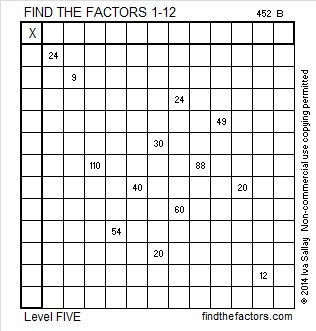Print the puzzles or type the factors on this excel file: 12 Factors 2014-12-29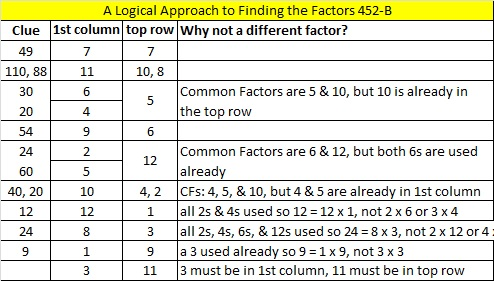# 339 and Level 4

• 339 is a composite number.
• Prime factorization: 339 = 3 x 113
• The exponents in the prime factorization are 1 and 1. Adding one to each and multiplying we get (1 + 1)(1 + 1) = 2 x 2 = 4. Therefore 339 has exactly 4 factors.
• Factors of 339: 1, 3, 113, 339
• Factor pairs: 339 = 1 x 339 or 3 x 113
• 339 has no square factors that allow its square root to be simplified. √339 ≈ 18.412Existential Spaghetti wrote a blog post a couple of years ago on New Year’s resolutions being yearly-chinese-finger-traps. People tend to choose resolutions that are “both time-consuming and often high-energy.” Ponder that as you solve this Level 4 Chinese Finger Trap Factoring Puzzle made especially for the New Year.Print the puzzles or type the factors on this excel file: 12 Factors 2014-12-29

Amazon.com sells these Chinese Finger Traps:# 338 Liebster Award or Not

• 338 is a composite number.
• Prime factorization: 338 = 2 x 13 x 13, which can be written 338 = 2 x 13²
• The exponents in the prime factorization are 1 and 2. Adding one to each and multiplying we get (1 + 1)(2 + 1) = 2 x 3  = 6. Therefore 338 has exactly 6 factors.
• Factors of 338: 1, 2, 13, 26, 169, 338
• Factor pairs: 338 = 1 x 338, 2 x 169, or 13 x 26
• Taking the factor pair with the largest square number factor, we get √338 = (√2)(√169) = 13√2 ≈ 18.385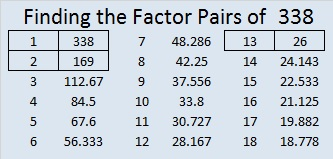Starguy recently nominated me for this blue-green Liebster award. Many bloggers have a no award policy, and I can certainly understand why: receiving an award is a bit of work if you accept it. The Liebster award has been around for a long time, and it has been said that just about every blogger has received it at one time or another.As these images demonstrate, some recipients have changed the way the award looks.
Since the logo has been changed multiple times by multiple people, I don’t feel bad having a somewhat rebellious attitude as I accept some of the qualifiers for the award while rejecting others. I’m not interested in sharing 11 random facts about myself, answering 11 questions, or coming up with 11 more to ask my nominees. I’m sure you will learn at least 11 things about me just reading this post anyway. (Random fact: I don’t like reading really long posts that go on forever, and this post will be long enough as it is.) If my nominees would like to write and answer questions, they can refer back to the questions asked in Starguy‘s post.

The idea of the Liebster award is to help bloggers get out of their comfort zones and discover new blogs, and I have done that. In fact several of the blogs I mention in this post I discovered within the last couple of weeks.

Rather than make a simple list of nominees, I’m going to do MORE than required and describe one of their noteworthy posts. Hopefully, the way that I do this will cause them to get a pingback notification because rebellious me is probably not going to inform them of their nominations any other way. Besides, if more people click on their links because I nominated them here, then the nomination will be meaningful. They will most likely notice when they view the referrers on their stat pages that Findthefactors is sending several people their way as well. Otherwise, this nomination won’t mean much. Nevertheless, please, click on their links. Here are my 11 nominees in no particular order:

1. Even though I am an English speaking grandma, I  listened only to Hungarian Christmas carols this year. Justkinga is a Hungarian teenage girl who listens to popular English music all year long. She takes TEN classes at a bilingual school, but somehow finds the time to write a delightful blog in ENGLISH regularly. I ADMIRE HER for writing in English even if there is an occasional grammar error or incorrect word choice. She also writes about Hungarian life and culture.
2. Established1962 wrote about an ingenious way to make Snakes and Ladders a game of decision making rather than mere chance. In the process, he made the game something even older players would enjoy playing while they observe some subtle mathematics.
3. Nebushumor wrote a very funny post about an extermination ad that featured an adorable Christmas mouse. Other times he’s written about Star Trek, funny family pet rabbit situations, classic cartoons, and comedy gags. Once a week he shares some kind of humorous statistics.
4. Solvemymaths shared a great link showing multiple ways to prove the Pythagorean theorem. The 3-4-5 triangle that accompanies his post is far more than a simple illustration. Solvemymaths routinely posts a variety of math problems, gifs, computer programs, and pictures that can help you think and learn to solve your own math problems.
5. Because I am a vegetarian, I was intrigued by notquitefrenchcuisine‘s old Hungarian family recipe for vegetarian burgers. I also love that she sprinkles a few Hungarian food words in her posts, too.
6. When I first read Puzzled Over’s Ages-of-three-daughters, I wasn’t sure how the last clue could possibly help me solve it. Maybe it will stump you at first, too, but it really can be solved without too much trouble.
7. One of the topics Classy Cheapskate blogs about is minimalism. She says a comfortable minimalist owns about 150 things. I can’t imagine that being possible for ANYONE right now, but I do want to head closer to that direction as does she.
8. I loved Paula Beardell Krieg’s instructions on how to fold paper to make an-equilateral-triangle and other basic shapes. Her method uses the straight edge of a piece of paper, but no compass is needed. Elementary school children can even make perfect squares or triangles using her methods.
9. Margarita Morris is a young adult author who has shared the complete writing process with her readers. Her books are well researched, written, and revised. She also shares her love of great classical music and can capture a scene in nature without writing a single word.
10. Resourceaholic scours the internet for resources that teach mathematics. Every one of her posts is loaded with as much information as this link. Your students will learn more if you read her blog, and chances are you will learn something, too!
11. Nerdinthebrain is a home-schooling parent who loves science and mathematics and sharing her lessons with us. Because of her, my grandson and I had a ton of fun testing the pH of different liquids using red cabbage water. She has MANY good ideas and products.
12. The Chaos Fairy was also nominated for the Liebster Award at the same time that I was so I’m not counting this as one of my 11. Nevertheless, I just had to recognize the DINOSAUR loving in this blog post.

# 337 What Will Be the Factors of 2015?

Since this is my 337th post, I’ll first give some information about the number 337, then I will predict the factors for 2015.

• 337 is a prime number.
• Prime factorization: 337 is prime.
• The exponent of prime number 337 is 1. Adding 1 to that exponent we get (1 + 1) = 2. Therefore 337 has exactly 2 factors.
• Factors of 337: 1, 337
• Factor pairs: 337 = 1 x 337
• 337 has no square factors that allow its square root to be simplified. √337 ≈ 18.358How do we know that 337 is a prime number? If 337 were not a prime number, then it would be divisible by at least one prime number less than or equal to √337 ≈ 18.358. Since 337 cannot be divided evenly by 2, 3, 5, 7, 11, 13, or 17, we know that 337 is a prime number.

Celebrate the New Year by giving this puzzle a try!

Print the puzzles or type the factors on this excel file: 12 Factors 2014-12-29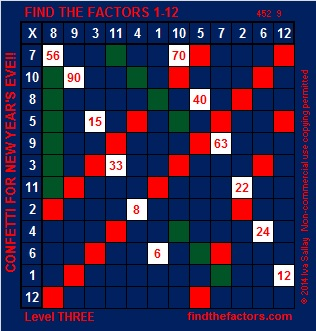On New Year’s Eve 2013 I predicted that the positive factors for 2014 would be 1, 2, 19, 38, 53, 106,1007, and 2014, and my predictions were 100% accurate!

On this last day of 2014, I boldly announce my predictions for the factors of the year 2015:

• The positive factors for 2015 will be 1, 5, 13, 31, 65, 155, 403, and 2015
• Some of these factors will occur in pairs: 1 and 2015, 5 and 403, 13 and 155, as well as 31 and 65.
• Unfortunately there will be some negative factors in 2015 as well. They will be -1, -5, -13, -31, -65, -155, -403, and -2015.

Whatever life throws your way, I wish you a happy, healthy, and prosperous 2015.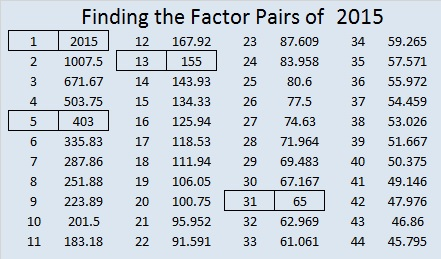# 336 and Level 2

### Today’s Puzzle:

The sixteen clues given in this puzzle are all you need to complete this multiplication table!Print the puzzles or type the factors on this excel file: 12 Factors 2014-12-29### Factors of 336:

• 336 is a composite number.
• Prime factorization: 336 = 2 x 2 x 2 x 2 x 3 x 7, which can be written 336 = (2^4) x 3 x 7
• The exponents in the prime factorization are 4, 1 and 1. Adding one to each and multiplying we get (4 + 1)(1 + 1)(1 + 1) = 5 x 2 x 2 = 20. Therefore 336 has exactly 20 factors.
• Factors of 336: 1, 2, 3, 4, 6, 7, 8, 12, 14, 16, 21, 24, 28, 42, 48, 56, 84, 112, 168, 336
• Factor pairs: 336 = 1 x 336, 2 x 168, 3 x 112, 4 x 84, 6 x 56, 7 x 48, 8 x 42, 12 x 28, 14 x 24 or 16 x 21
• Taking the factor pair with the largest square number factor, we get √336 = (√16)(√21) = 4√21 ≈ 18.330### Sum-Difference Puzzles:

84 has six factor pairs. One of those factor pairs adds up to 25, and another one subtracts to 25. Can you determine what those factor pairs are to solve the first puzzle below?

336 has ten factor pairs. One of them adds up to 50, and a different one subtracts to 50. If you can identify those factor pairs, then you can solve the second puzzle!The second puzzle is really just the first puzzle in disguise. Why would I say that?

# 335 and Level 1

• 335 is a composite number.
• Prime factorization: 335 = 5 x 67
• The exponents in the prime factorization are 1 and 1. Adding one to each and multiplying we get (1 + 1)(1 + 1) = 2 x 2 = 4. Therefore 335 has exactly 4 factors.
• Factors of 335: 1, 5, 67, 335
• Factor pairs: 335 = 1 x 335 or 5 x 67
• 335 has no square factors that allow its square root to be simplified. √335 ≈ 18.303If you can multiply, divide and use a little logic, you should easily be able to complete this multiplication table puzzle.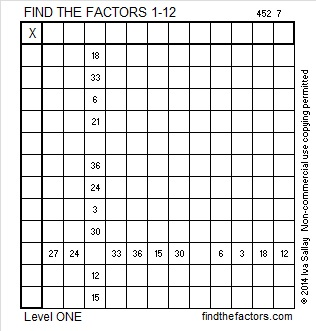Print the puzzles or type the factors on this excel file: 12 Factors 2014-12-29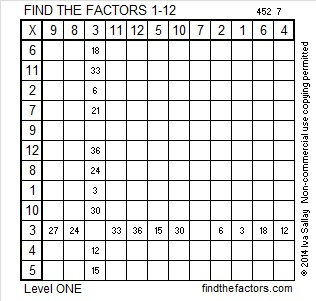# 334 and Level 6

• 334 is a composite number.
• Prime factorization: 334 = 2 x 167
• The exponents in the prime factorization are 1 and 1. Adding one to each and multiplying we get (1 + 1)(1 + 1) = 2 x 2 = 4. Therefore 334 has exactly 4 factors.
• Factors of 334: 1, 2, 167, 334
• Factor pairs: 334 = 1 x 334 or 2 x 167
• 334 has no square factors that allow its square root to be simplified. √334 ≈ 18.276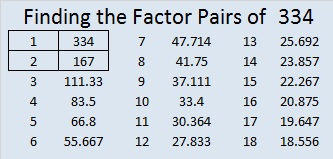Solving this Level 6 puzzle can be a little tricky: Is 1 or 2 the common factor for 6 and 8? Is 3 or 6 the common factor for 12 and 30? Is 4 or 8 the common factor for 40 and 16? In each case only one of those choices will make this puzzle work as a multiplication table? Can you figure out what those choices should be? Use logic to find the correct solution, not trial and error.Print the puzzles or type the factors on this excel file:  10 Factors 2014-12-22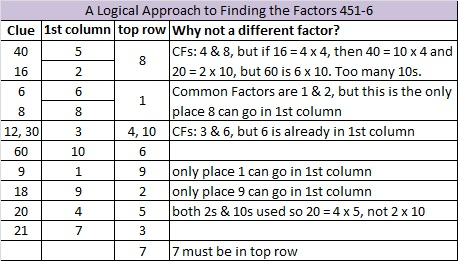# 333 and Level 5

• 333 is a composite number.
• Prime factorization: 333 = 3 x 3 x 37, which can be written 333 = 3² x 37
• The exponents in the prime factorization are 2 and 1. Adding one to each and multiplying we get (2 + 1)(1 + 1) = 3 x 2  = 6. Therefore 333 has exactly 6 factors.
• Factors of 333: 1, 3, 9, 37, 111, 333
• Factor pairs: 333 = 1 x 333, 3 x 111, or 9 x 37
• Taking the factor pair with the largest square number factor, we get √333 = (√9)(√37) = 3√37 ≈ 18.248Can the eleven clues in this puzzle help you to complete this multiplication table?Print the puzzles or type the factors on this excel file:  10 Factors 2014-12-22# 332 and a Christmas Star

• 332 is a composite number.
• Prime factorization: 332 = 2 x 2 x 83 which can be written 2² x 83
• The exponents in the prime factorization are 1 and 1. Adding one to each and multiplying we get (2 + 1)(1 + 1) = 3 x 2 = 6. Therefore 332 has exactly 6 factors.
• Factors of 332: 1, 2, 4, 83, 166, 332
• Factor pairs: 332 = 1 x 332, 2 x 166, or 4 x 83
• Taking the factor pair with the largest square number factor, we get √332 = (√4)(√83) = 2√83 ≈ 18.221Merry Christmas! This is a rather easy level 5 puzzle so I’m sharing it instead of a level 4 puzzle today.Print the puzzles or type the factors on this excel file:  10 Factors 2014-12-22

The Night Wind excitedly asked a little lamb and the whole world, “Do you see what I see?” when it saw “a star, a star, dancing in the night” after the Christ child was born. Click here to read the complete lyrics of “Do You Hear What I Hear.”# 331 and Hockey Sticks

• 331 is a prime number.
• Prime factorization: 331 is prime.
• The exponent of prime number 331 is 1. Adding 1 to that exponent we get (1 + 1) = 2. Therefore 331 has exactly 2 factors.
• Factors of 331: 1, 331
• Factor pairs: 331 = 1 x 331
• 331 has no square factors that allow its square root to be simplified. √331 ≈ 18.193How do we know that 331 is a prime number? If 331 were not a prime number, then it would be divisible by at least one prime number less than or equal to √331 ≈ 18.193. Since 331 cannot be divided evenly by 2, 3, 5, 7, 11, 13, or 17, we know that 331 is a prime number.Print the puzzles or type the factors on this excel file:  10 Factors 2014-12-22

Today’s puzzle looks like a hockey stick. Hockey sticks remind me not only of the obvious winter sport but also of the Twelve Days of Christmas and Pascal’s triangle.

Dimacs.rutgers.edu explains quite nicely how a hockey stick in Pascal’s triangle can give you the total number of gifts received after one day, two days, three days, and so on. Look at the green and red hockey stick with bold black numbers in this illustration of Pascal’s triangle:If someone gave you one partridge every day for 12 days, two turtle doves every day for 11 days, three French hens every day for 10 days, etc, etc, and etc, then you would receive 364 gifts. (364 is so easy to remember because it is one less than the number of days in a year.)

A Logical Approach to FIND THE FACTORS: Find the column or row with two clues and find their common factor. Write the corresponding factors in the factor column (1st column) and factor row (top row).  Because this is a level three puzzle, you have now written a factor at the top of the factor column. Continue to work from the top of the factor column to the bottom, finding factors and filling in the factor column and the factor row one cell at a time as you go.